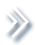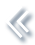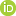####ArchivesReview Articles

## Efficient GMM estimation with singular system of moment conditions

Zhiguo Xiao

Department of Statistics, School of Management, Fudan University, Shanghai, People's Republic of Chinazhiguo_xiao@fudan.edu.cn

Pages 172-178 | Received 21 Apr. 2019, Accepted 05 Aug. 2019, Published online: 23 Aug. 2019,
• Abstract
• References
• Citations

Abstract

Standard generalised method of moments (GMM) estimation was developed for nonsingular system of moment conditions. However, many important economic models are characterised by singular system of moment conditions. This paper shows that efficient GMM estimation of such models can be achieved by using the reflexive generalised inverses, in particular the Moore–Penrose generalised inverse, of the variance matrix of the sample moment conditions as the weighting matrix. We provide a consistent estimator of the optimal weighting matrix and establish its consistency. Potential issues of using generalised inverse and some remedies are also discussed.

## References

1. Abadir, K. M., & Magnus, J. R. (2005). Matrix algebra. New York: Cambridge University Press. [Crossref], [Google Scholar]
2. Alessi, L., Barigozzi, M., & Capasso, M. (2011). Non-fundamentalness in structural econometric models: A review. International Statistical Review79, 16–47. doi: 10.1111/j.1751-5823.2011.00131.x [Crossref][Web of Science ®], [Google Scholar]
3. Bapat, R. B. (2012). Linear algebra and linear models (3rd ed.). London: Springer-Verlag. [Crossref], [Google Scholar]
4. Barten, A. (1969). Maximum likelihood estimation of a complete system of demand equations. European Economic Review1, 7–73. doi: 10.1016/0014-2921(69)90017-8 [Crossref][Web of Science ®], [Google Scholar]
5. Barten, A. (1977). The system of consumer demand function approach: A review. Econometrica45, 23–51. doi: 10.2307/1913286 [Crossref][Web of Science ®], [Google Scholar]
6. Berndt, E. R., & Christensen, L. R. (1974). Testing for the existence of a consistent aggregate index of labor inputs. American Economics Review64, 391–403. [Web of Science ®], [Google Scholar]
7. Bierens, H. J. (2007). Econometric analysis of linearized singular dynamic stochastic general equilibrium models. Journal of Econometrics136, 595–627. doi: 10.1016/j.jeconom.2005.11.008 [Crossref][Web of Science ®], [Google Scholar]
8. Bierens, H. J., & Swanson, N. R. (2000). The econometric consequences of the ceteris paribus condition in economic theory. Journal of Econometrics95, 223–253. doi: 10.1016/S0304-4076(99)00038-X [Crossref][Web of Science ®], [Google Scholar]
9. Biørn, E. (2000). Panel data with measurement errors: Instrumental variables and GMM procedures combining levels and differences. Econometric Reviews19(4), 391–424. doi: 10.1080/07474930008800480 [Taylor & Francis Online], [Google Scholar]
10. Biørn, E., & Klette, T. (1998). Panel data with errors-in-variables: Essential and redundant orthogonal conditions in GMM-estimation. Economics Letters59, 275–282. doi: 10.1016/S0165-1765(98)00053-6 [Crossref][Web of Science ®], [Google Scholar]
11. Chen, Y., Hong, C., & Riley, R. D. (2014). An alternative pseudolikelihood method for multivariate random-effects meta-analysis. Statistics in Medicine34(3), 361–380. doi: 10.1002/sim.6350 [Crossref][Web of Science ®], [Google Scholar]
12. Dhrymes, P. J. (1962). On devising unbiased estimators for the parameters of a Cobb–Douglas production function. Econometrica30, 297–304. doi: 10.2307/1910218 [Crossref][Web of Science ®], [Google Scholar]
13. Dhrymes, P. J., & Schwarz, S. (1987). On the existence of generalized inverse estimators in a singular system of equations. Journal of Forecasting6, 181–192. doi: 10.1002/for.3980060304 [Crossref][Web of Science ®], [Google Scholar]
14. Fampa, M., & Lee, J. (2018). On sparse reflexive generalized inverses. Operations Research Letters46(6), 605–610. doi: 10.1016/j.orl.2018.09.005 [Crossref][Web of Science ®], [Google Scholar]
15. Hall, A. (2005). Generalized method of moments. Oxford University Press: New York. [Google Scholar]
16. Hansen, L. (1982). Large sample properties of generalized method of moments estimators. Econometrica50, 1029–1054. doi: 10.2307/1912775 [Crossref][Web of Science ®][Google Scholar]
17. Hansen, B., & West, K. (2002). Generalized method of moments and macroeconomics. Journal of Business & Economic Statistics20, 460–469. doi: 10.1198/073500102288618603 [Taylor & Francis Online][Web of Science ®], [Google Scholar]
18. Haupt, H., & Oberhofer, W. (2006). Generalized adding-up in systems of regression equations. Economics Letters92, 263–269. doi: 10.1016/j.econlet.2006.03.001 [Crossref][Web of Science ®], [Google Scholar]
19. Ireland, P. N. (2004). A method for taking models to the data. Journal of Economic Dynamics and Control28, 1205–1226. doi: 10.1016/S0165-1889(03)00080-0 [Crossref][Web of Science ®], [Google Scholar]
20. Kreijger, R. G., & Neudecker, H. (1977). Exact linear restrictions on parameters in the general linear model with a singular covariance matrix. Journal of the American Statistical Association72, 430–432. doi: 10.1080/01621459.1977.10481014 [Taylor & Francis Online][Web of Science ®], [Google Scholar]
21. Lai, H. (2008). Maximum likelihood estimation of singular systems of equations. Economics Letters99, 51–54. doi: 10.1016/j.econlet.2007.05.027 [Crossref][Web of Science ®], [Google Scholar]
22. Leeper, E. M., Walker, T. B., & Yang, S.-C. S. (2013). Fiscal foresight and information flows. Econometrica81, 1115–1145. doi: 10.3982/ECTA8337 [Crossref][Web of Science ®], [Google Scholar]
23. Mountford, A., & Uhlig, H. (2009). What are the effects of fiscal policy shocks?. Journal of Applied Econometrics24, 960–992. doi: 10.1002/jae.1079 [Crossref][Web of Science ®], [Google Scholar]
24. Penrose, R. (1955). A generalized inverse for matrices. Mathematical Proceedings of the Cambridge Philosophical Society51, 406–413. doi: 10.1017/S0305004100030401 [Crossref], [Google Scholar]
25. Rao, C. R. (1972). Alternative econometric models of sales advertising relationships. Journal of Marketing Research9, 171–181. doi: 10.1177/002224377200900209 [Crossref][Web of Science ®], [Google Scholar]
26. Rao, C. R., & Mitra, S. K. (1971). Generalized inverse of matrices and its applications. John Wiley & Sons, Inc.: New York. [Google Scholar]
27. Riley, R., Abrams, K., Lambert, P., Sutton, A., & Thompson, J. (2007). An evaluation of bivariate random-effects meta-analysis for the joint synthesis of two correlated outcomes. Statistics in Medicine26(1), 78–97. doi: 10.1002/sim.2524 [Crossref][Web of Science ®], [Google Scholar]
28. Schneeweiss, H. (2014). The linear GMM model with singular covariance matrix due to the elimination of a nuisance parameter. Technical Report 165, Dept. Statistics, Univ. Munich. [Google Scholar]
29. Stewart, G. W. (1969). On the continuity of the generalized inverse. SIAM Journal of Applied Mathematics17, 33–45. doi: 10.1137/0117004 [Crossref][Web of Science ®], [Google Scholar]
30. Theil, H. (1971). Principle of econometrics. John Wiley & Sons: New York. [Google Scholar]
31. Velasco, C., & Lobato, I. N. (2018). Frequency domain minimum distance inference for possibly noninvertible and noncausal ARMA models. The Annals of Statistics46(2), 555–579. doi: 10.1214/17-AOS1560 [Crossref][Web of Science ®], [Google Scholar]
32. Wansbeek, T. J. (2001). GMM Estimation in panel data models with measurement error. Journal of Econometrics104, 259–268. doi: 10.1016/S0304-4076(01)00079-3 [Crossref][Web of Science ®], [Google Scholar]
33. Weiss, D (1968). Determinants of market share. Journal of Marketing Research5, 290–295. doi: 10.1177/002224376800500307 [Crossref][Web of Science ®], [Google Scholar]
34. White, H. (1986). Instrumental variables analogs of generalized least squares estimators. Advances in Statistical Analysis and Statistical Computing1, 173–227. [Google Scholar]
35. Xiao, Z. (2008). Generalized inverses in GMM estimation with redundant moment conditions. In Topics in Generalized Method of Moments Estimation with Application to Panel Data with Measurement Error, University of Wisconsin-Madison PhD thesis. [Google Scholar]
36. Xiao, Z., Shao, J., & Palta, M. (2010a). GMM in linear regression for longitudinal data with multiple covariates measured with error. Journal of Applied Statistics37, 791–805. doi: 10.1080/02664760902890005 [Taylor & Francis Online][Web of Science ®], [Google Scholar]
37. Xiao, Z., Shao, J., & Palta, M. (2010b). Instrumental variable and GMM estimation of panel data with measurement error. Statistica Sinica20(4), 1725–1747. [Web of Science ®], [Google Scholar]
38. Xiao, Z., Shao, J., Xu, R., & Palta, M. (2007). Efficiency of GMM estimation in panel data models with measurement error. Sankhya: The Indian Journal of Statistics69, 101–118. [Google Scholar]
39. Xu, L., Fampa, M., & Lee, J. (2019). Aspects of symmetry for sparse reflexive generalized inverses. arXiv:1903.05744v1[math.OC]. [Google Scholar]